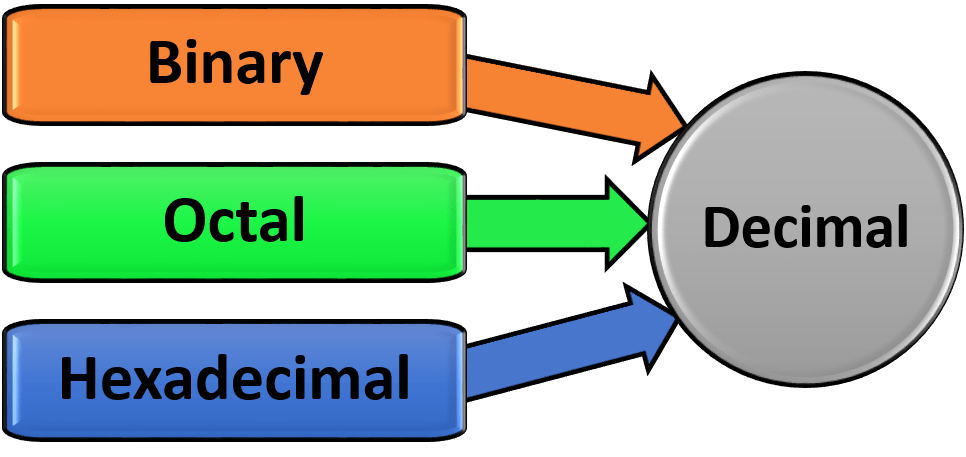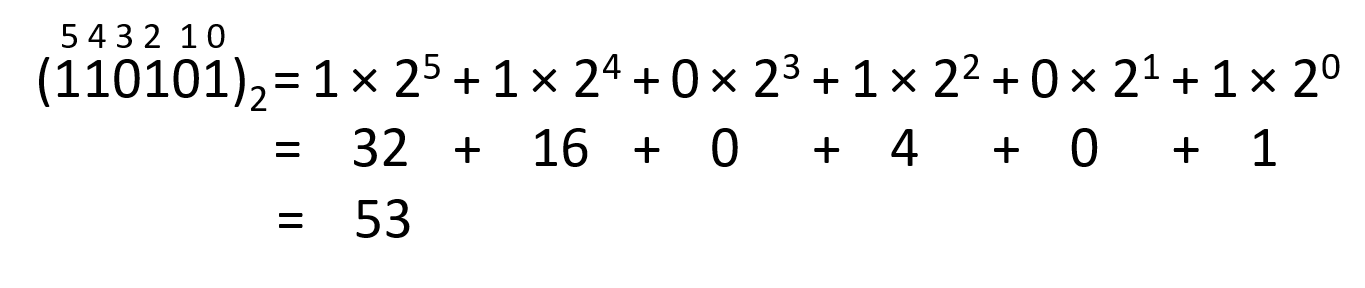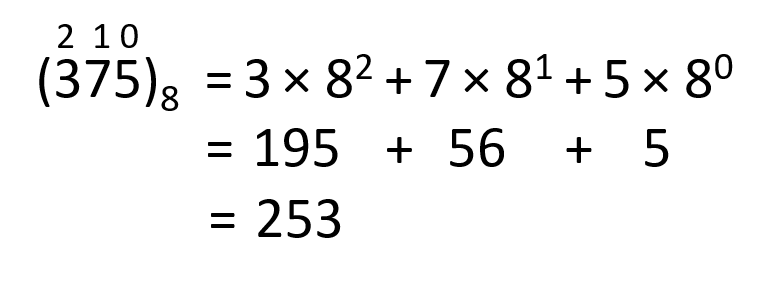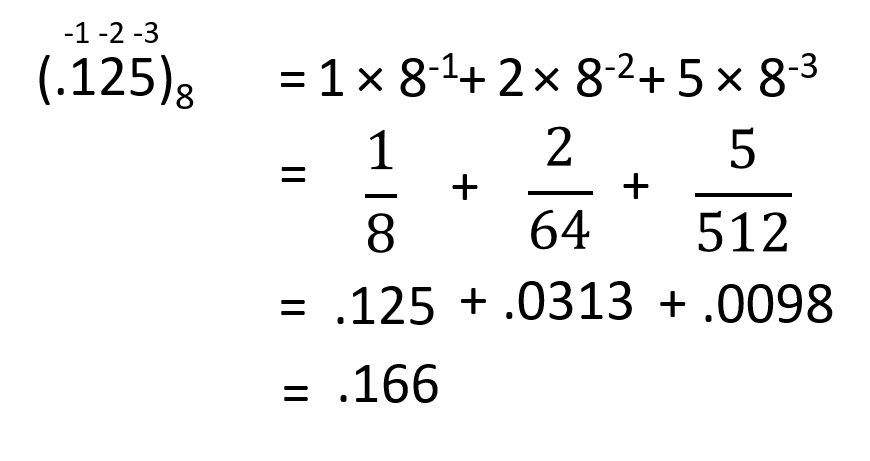# Third Chapter Lesson-3: Conversion of Binary, Octal & Hexadecimal number into Decimal number.

## At the end of this lesson-

• 1. You will be able to convert a binary number into decimal number.
• 2. You will be able to convert a octal number into decimal number.
• 3. You will be able to convert a hexadecimal number into decimal number.

## Other Base to Decimal Number System### Same Rules for Both Integer and Fractional Number-

Step-1: Multiply each digit of the given number by their positional value.

Positional value of a digit = (base of given number)position of the digit

[ In integer number, position of the digit starts from 0 (right to left) and in fractional number position of the digit starts from -1 (left to right). ]

Step-2: Perform the addition of all terms to obtain the number in base 10.

Obtained Summation will be equivalent decimal number to the given number.

## Binary number into decimal number:

Example: Convert (110101)2 to decimal number system.So,(110101)2 = (53)10

Example: Convert (.1010)2  to decimal number system.

####So, (.1010)2 = (.625)10

• Convert (101010.0101)2  to decimal number system.
• Convert (1100011.10101)2  to decimal number system.

## Octal number into decimal number:

Example: Convert (375)8 to decimal number system.So, (375)8 = (253)10

Example: Convert (.125)8 to decimal number system.

#So, (.125)8 = (.166)10

• Convert (567.247)8 to decimal number system.
• Convert (3702.6040)8 to decimal number system.

## Hexadecimal number into decimal number:

Example: Convert (3FC)16 to decimal number system.So, (3FC)16 = (1020)10

Example: Convert (.2B)16 to decimal number system.

##So, (.2B)16 = (.168)10

• Convert (7A6B.9B8)16 to decimal number system.
• Convert (89A.10F)16 to decimal number system.

## Lesson Evaluation-

Knowledge Based Questions:

Comprehension Based Questions:

Creative Questions:

According to the stem answer the following questions:

The professor of ICT was teaching the number system in the classroom. When asked about Imran’s half-yearly and annual test scores for his ICT subject, he said he got (37)8 marks in half-yearly and (3F)16 marks in annual examination. When the other students asked Sir if they did not understand what it meant, Sir explained the details.

c) Convert the marks that Imran obtained in half yearly exam into Decimal number.

d) How much more or less is Imran’s annual test score from (72)10? Argue for your answer.

Multiple Choice Questions:

According to the stem answer the question no-1:

ICT teacher Shafiq sir has written (77)8 on the Board.

1. What will be decimal number of the stimulas number?

a) 56    b) 63    c) 64    d) 77

2. Which one is the equivalent decimal number of (B5D)16 ?

a) 2909    b) 3909    c) 1909    d) 4909

3. The price of a book is 1001011 in binary, what will be in decimal?

a) 70     b) 75      c) 78   d) 80

According to the stem answer the question no – 4:

The student has written his roll (1001)2 as the teacher asked to write.

4. What will be the student’s roll in decimal?

a) 5      b) 9     c) 16     d) 17

Written by,